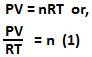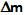## Thursday, January 14, 2010

### Irodov Problem 2.1

From the ideal gas law equation we know that,Here P,V and T are the pressure, volume and temperature of the gas. n is the number of moles of the gas and R the gas constant. In our problem since V and T remain unchanged we have,In (2),is the mass of gas that leaked out and M is the molar mass of the gas. We also know the the density of the gas at atmospheric pressure and the same temperature (I guess this is what Irodov means by standard conditions) and so we can write,